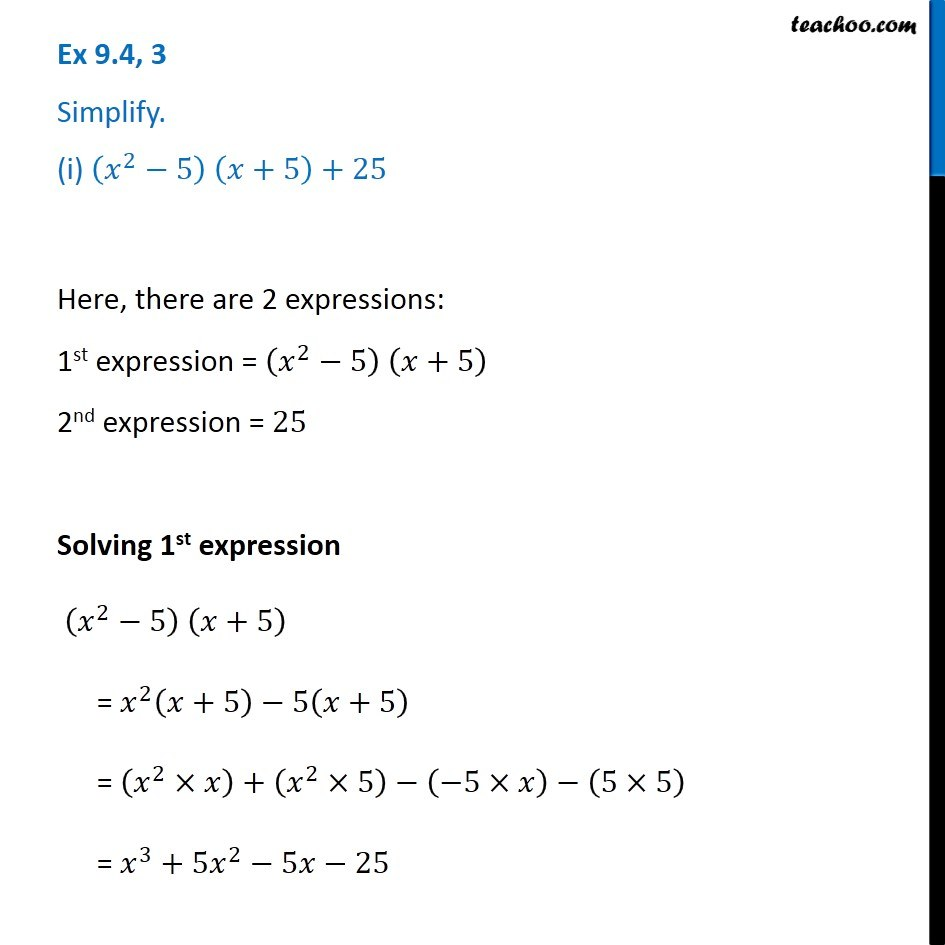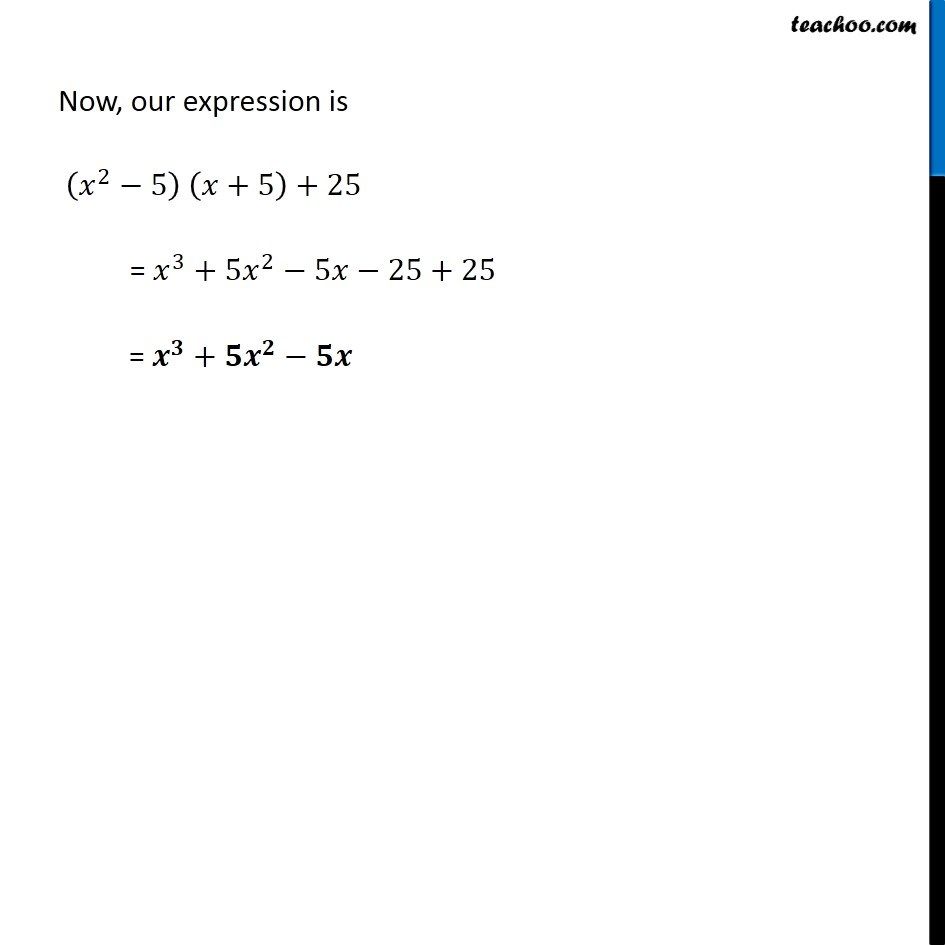Subscribe to our Youtube Channel - https://you.tube/teachoo

1. Chapter 9 Class 8 Algebraic Expressions and Identities
2. Concept wise
3. Multiplication of Polynoimals by Polynomials

Transcript

Ex 9.4, 3 Simplify. (i) (𝑥^2−5) (𝑥+5)+25 Here, there are 2 expressions: 1st expression = (𝑥^2−5) (𝑥+5) 2nd expression = 25 Solving 1st expression (𝑥^2−5) (𝑥+5) = 𝑥^2 (𝑥+5)−5(𝑥+5) = (𝑥^2×𝑥)+(𝑥^2×5)−(−5×𝑥)−(5×5) = 𝑥^3+5𝑥^2−5𝑥−25 Now, our expression is (𝑥^2−5) (𝑥+5)+25 = 𝑥^3+5𝑥^2−5𝑥−25+25 = 𝒙^𝟑+𝟓𝒙^𝟐−𝟓𝒙

Multiplication of Polynoimals by Polynomials

About the AuthorDavneet Singh
Davneet Singh is a graduate from Indian Institute of Technology, Kanpur. He has been teaching from the past 9 years. He provides courses for Maths and Science at Teachoo.